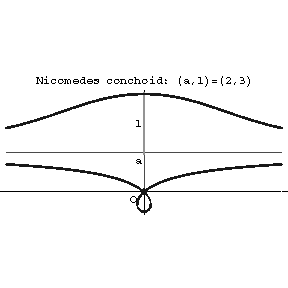# Nicomedes conchoid

Jump to: navigation, search

A plane algebraic curve of order 4 whose equation in Cartesian rectangular coordinates has the form

$$(x^2+y^2)(x-a)^2-l^2x^2=0;$$

and in polar coordinates

$$\rho=\frac{a}{\sin\psi}\pm l.$$Figure: n066620a

Outer branch (see Fig.). Asymptote $x=a$. Two points of inflection, $B$ and $C$.

Inner branch. Asymptote $x=a$. The coordinate origin is a double point whose character depends on the values of $a$ and $l$. For $l<a$ it is an isolated point and, in addition, the curve has two points of inflection, $E$ and $F$; for $l>a$ it is a node; for $l=a$ it is a cusp. The curve is a conchoid of the straight line $x=a$.

The curve is named after Nicomedes (3rd century B.C.), who used it to solve the problem of trisecting an angle.